#### An eye's view

Figure 3.14 shows a virtual eye that is looking down the negative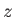axis. It is placed in this way so that from the eye's perspective,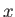increases to the right and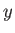is upward. This corresponds to familiar Cartesian coordinates. The alternatives would be: 1) to face the eye in the positivedirection, which makes the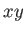coordinates appear backwards, or 2) reverse theaxis, which would unfortunately lead to a left-handed coordinate system. Thus, we have made an odd choice that avoids worse complications.

Suppose that the eye is an object model that we want to place into the virtual world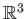at some position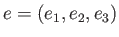and orientation given by the matrix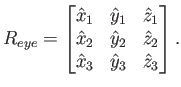(3.35)

If the eyeball in Figure 3.14 were made of triangles, then rotation by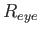and translation by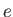would be applied to all vertices to place it in.

This does not, however, solve the problem of how the virtual world should appear to the eye. Rather than moving the eye in the virtual world, we need to move all of the models in the virtual world to the eye's frame of reference. This means that we need to apply the inverse transformation. The inverse rotation is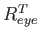, the transpose of. The inverse ofis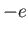. Applying (3.26) results in the appropriate transform: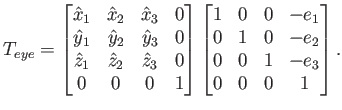(3.36)

Note that, as shown in (3.35), has been transposed and placed into the left matrix above. Also, the order of translation and rotation have been swapped, which is required for the inverse, as mentioned in Section 3.2.

Following Figure 3.4, there are two possible interpretations of (3.36). As stated, this could correspond to moving all of the virtual world models (corresponding to Figure 3.4(b)). A more appropriate interpretation in the current setting is that the virtual world's coordinate frame is being moved so that it matches the eye's frame from Figure 3.14. This corresponds to the case of Figure 3.4(c), which was not the appropriate interpretation in Section 3.2.

Steven M LaValle 2020-11-11